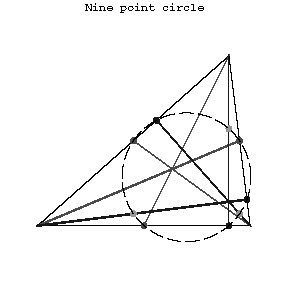# Nine-point circle

2010 Mathematics Subject Classification: Primary: 51M15 [MSN][ZBL]

Euler circle, Feuerbach circle

A circle whose periphery contains the midpoints of the sides of a triangle, the bases of its altitudes, and the midpoints of the segment connecting the orthocentre of the triangle with the vertices. Its radius is equal to one-half of the radius of the circle circumscribed about the triangle. The nine-point circle of a triangle is tangent to the circle inscribed in it and to the three escribed circles. Let \$H\$ be the orthocentre of a non-equilateral triangle, let \$T\$ be the centre of gravity, let \$O\$ be the centre of the circumscribed circle and let \$E\$ be the centre of the nine-point circle. The points \$H,T,O,E\$ then lie on a straight line (the Euler line), \$E\$ being the midpoint of the segment \$HO\$, and the pair of points \$H,T\$ harmonically subdivides the pair of points \$O,E\$.Figure: n066750a

How to Cite This Entry:
Nine-point circle. Encyclopedia of Mathematics. URL: http://encyclopediaofmath.org/index.php?title=Nine-point_circle&oldid=39655
This article was adapted from an original article by V.T. Bazylev (originator), which appeared in Encyclopedia of Mathematics - ISBN 1402006098. See original article